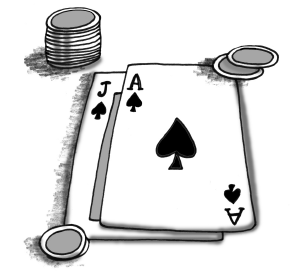### Home > CCG > Chapter 11 > Lesson 11.2.1 > Problem11-87

11-87.

In the card game called “Twenty-One,” two cards are dealt from a randomly shuffled deck of playing cards. The player’s goal is to get the sum of his or her cards to be as close (or equal) to $21$ as possible, without going over $21$. To establish the sample space for this problem, you need to think of choosing two from a set of $52$.

1.How many ways are there to be dealt two cards?

$_{n}C_{r}=\frac{n!}{r!(n-r!)}$

1. The tens, jacks, queens, and kings all have a value of $10$ points. There are four of each in a standard deck. What is the probability of being dealt two ten-valued cards?

How many ways are there to select two cards from the set of ten-valued cards?

Divide this number by the total number of $2$-card hands.

2. If you did not already do so, write your solution to part (b) in the form $\frac {_a C _ { b } } {_d C _ { e } }$.

$\frac{_{2}C_{1}6}{_{2}C_{5}2}$

3. What is the probability (as a percent) of being dealt two face cards? (Face cards are Kings, Queens, and Jacks, that is, the cards that have faces.) Also write your answer as $\frac {_a C _ { b } } {_d C _ { e } }$.

See part (b).

4. In “Twenty-One” an Ace can count as $1$ point or $11$ points. The deal is called “soft” when the Ace is counted as $11$ points. What is the probability of being dealt a “soft $21$,” that is, a card worth ten points and an Ace?

See part (c).